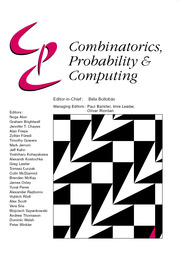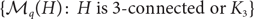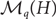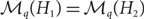Home
Hostname: page-component-684899dbb8-x64cq Total loading time: 0.313 Render date: 2022-05-19T00:22:39.867Z Has data issue: true Feature Flags: { "shouldUseShareProductTool": true, "shouldUseHypothesis": true, "isUnsiloEnabled": true, "useRatesEcommerce": false, "useNewApi": true }Combinatorics, Probability and Computing

# On minimal Ramsey graphs and Ramsey equivalence in multiple colours

Published online by Cambridge University Press:  09 March 2020

*
*Corresponding author. Email: a.liebenau@unsw.edu.au

## Abstract

For an integer q ⩾ 2, a graph G is called q-Ramsey for a graph H if every q-colouring of the edges of G contains a monochromatic copy of H. If G is q-Ramsey for H yet no proper subgraph of G has this property, then G is called q-Ramsey-minimal for H. Generalizing a statement by Burr, Nešetřil and Rödl from 1977, we prove that, for q ⩾ 3, if G is a graph that is not q-Ramsey for some graph H, then G is contained as an induced subgraph in an infinite number of q-Ramsey-minimal graphs for H as long as H is 3-connected or isomorphic to the triangle. For such H, the following are some consequences.

• For 2 ⩽ r < q, every r-Ramsey-minimal graph for H is contained as an induced subgraph in an infinite number of q-Ramsey-minimal graphs for H.

• For every q ⩾ 3, there are q-Ramsey-minimal graphs for H of arbitrarily large maximum degree, genus and chromatic number.

• The collection$\{\mathcal M_q(H) \colon H \text{ is 3-connected or } K_3\}$ forms an antichain with respect to the subset relation, where$\mathcal M_q(H)$ denotes the set of all graphs that are q-Ramsey-minimal for H.

We also address the question of which pairs of graphs satisfy$\mathcal M_q(H_1)=\mathcal M_q(H_2)$ , in which case H1 and H2 are called q-equivalent. We show that two graphs H1 and H2 are q-equivalent for even q if they are 2-equivalent, and that in general q-equivalence for some q ⩾ 3 does not necessarily imply 2-equivalence. Finally we indicate that for connected graphs this implication may hold: results by Nešetřil and Rödl and by Fox, Grinshpun, Liebenau, Person and Szabó imply that the complete graph is not 2-equivalent to any other connected graph. We prove that this is the case for an arbitrary number of colours.

## MSC classification

Type
Paper
Information
Combinatorics, Probability and Computing , July 2020 , pp. 537 - 554

## Access options

Get access to the full version of this content by using one of the access options below. (Log in options will check for institutional or personal access. Content may require purchase if you do not have access.)

## Footnotes

Supported by an ARC Decra Fellowship. Previously at Monash University where this research was partly carried out.

## References

Axenovich, M., Rollin, J. and Ueckerdt, T. (2017) Conditions on Ramsey nonequivalence. J. Graph Theory 86 159192.Google Scholar
Balister, P., Lehel, J. and Schelp, R. (2006) Ramsey unsaturated and saturated graphs. J. Graph Theory 51 2232.CrossRefGoogle Scholar
Bloom, T. and Liebenau, A. (2018) Ramsey equivalence of Kn and Kn + Kn-1. Electron. J. Combin. 25 P3.4.Google Scholar
Bodkin, C. (2016) Folkman numbers and a conjecture in Ramsey theory. Master’s thesis, FU Berlin.Google Scholar
Bollobás, B., Donadelli, J., Kohayakawa, Y. and Schelp, R. (2001) Ramsey minimal graphs. J. Brazil. Comput. Soc. 7 2737.CrossRefGoogle Scholar
Burr, S., Erdős, P., Faudree, R., Rousseau, C. and Schelp, R. (1981) Ramsey-minimal graphs for star-forests. Discrete Math. 33 227237.Google Scholar
Burr, S., Erdős, P. and Lovász, L. (1976) On graphs of Ramsey type. Ars Combin. 1 167190.Google Scholar
Burr, S., Faudree, R. and Schelp, R. (1977) On Ramsey-minimal graphs. In Proc. 8th Southeastern Conference on Combinatorics, Graph Theory and Computing, pp. 115124.Google Scholar
Burr, S., Nešetřil, J. and Rödl, V. (1985) On the use of senders in generalized Ramsey theory for graphs. Discrete Math. 54 113.Google Scholar
Conlon, D. (2009) A new upper bound for diagonal Ramsey numbers. Ann. of Math. 170 941960.Google Scholar
Erdős, P. (1947) Some remarks on the theory of graphs. Bull. Amer. Math. Soc. 53 292294.CrossRefGoogle Scholar
Erdős, P. and Szekeres, G. (1935) A combinatorial problem in geometry. Compositio Math. 2 463470.Google Scholar
Faudree, R. (2018) Ramsey minimal graphs for forests. Ars Combin 31 117124.Google Scholar
Folkman, J. (1970) Graphs with monochromatic complete subgraphs in every edge coloring. SIAM J. Appl. Math. 18 1924.Google Scholar
Fox, J., Grinshpun, A., Liebenau, A., Person, Y. and Szabó, T. (2014) What is Ramsey-equivalent to a clique? J. Combin. Theory Ser. B 109 120133.Google Scholar
Fox, J., Grinshpun, A., Liebenau, A., Person, Y. and Szabó, T. (2016) On the minimum degree of minimal Ramsey graphs for multiple colours. J. Combin. Theory Ser. B 120 6482.Google Scholar
Fox, J. and Lin, K. (2006) The minimum degree of Ramsey-minimal graphs. J. Graph Theory 54 167177.CrossRefGoogle Scholar
Graham, R. and Rödl, V. (1987) Numbers in Ramsey theory. Surveys Combin. 123 111153.Google Scholar
Guo, H. and Warnke, L. (2020) Packing nearly optimal Ramsey R(3, t) graphs. https://doi.org/10.1007/s00493-019-3921-7 CrossRefGoogle Scholar
Hàn, H., Rödl, V. and Szabó, T. (2018) Vertex Folkman numbers and the minimum degree of minimal Ramsey graphs. SIAM J. Discrete Math. 32 826838.CrossRefGoogle Scholar
Lin, S. (1972) On Ramsey numbers and K r -coloring of graphs. J. Combin. Theory Ser. B 12 8292.CrossRefGoogle Scholar
Łuczak, T. (1994) On Ramsey minimal graphs. Electron. J. Combin 1 R4.CrossRefGoogle Scholar
Nešetřil, J. and Rödl, V. (1976) The Ramsey property for graphs with forbidden complete subgraphs. J. Combin. Theory Ser. B 20 243249.Google Scholar
Ramsey, F. (1930) On a problem of formal logic. Proc. London Math. Soc. 30 264286.CrossRefGoogle Scholar
Rödl, V. and Ruciński, A. (1995) Threshold functions for Ramsey properties. J. Amer. Math. Soc. 8 917942.CrossRefGoogle Scholar
Rödl, V. and Siggers, M. (2008) On Ramsey minimal graphs. SIAM J. Discrete Math. 22 467488.CrossRefGoogle Scholar
Spencer, J. (1977) Asymptotic lower bounds for Ramsey functions. Discrete Math. 20 6976.CrossRefGoogle Scholar
Szabó, T., Zumstein, P. and Zürcher, S. (2010) On the minimum degree of minimal Ramsey graphs. J. Graph Theory 64 150164.Google Scholar
Thomason, A. (1988) An upper bound for some Ramsey numbers. J. Graph Theory 12 509517.CrossRefGoogle Scholar
1
Cited by

# Save article to Kindle

Note you can select to save to either the @free.kindle.com or @kindle.com variations. ‘@free.kindle.com’ emails are free but can only be saved to your device when it is connected to wi-fi. ‘@kindle.com’ emails can be delivered even when you are not connected to wi-fi, but note that service fees apply.

Find out more about the Kindle Personal Document Service.

On minimal Ramsey graphs and Ramsey equivalence in multiple colours
Available formats
×

# Save article to Dropbox

To save this article to your Dropbox account, please select one or more formats and confirm that you agree to abide by our usage policies. If this is the first time you used this feature, you will be asked to authorise Cambridge Core to connect with your Dropbox account. Find out more about saving content to Dropbox.

On minimal Ramsey graphs and Ramsey equivalence in multiple colours
Available formats
×

# Save article to Google Drive

To save this article to your Google Drive account, please select one or more formats and confirm that you agree to abide by our usage policies. If this is the first time you used this feature, you will be asked to authorise Cambridge Core to connect with your Google Drive account. Find out more about saving content to Google Drive.

On minimal Ramsey graphs and Ramsey equivalence in multiple colours
Available formats
×
×